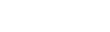# In this article, we will discuss some of the console methods which are available to use and which can increase the productivity of web developers.Himanshu Pareek

4 months ago | 3 min read
FollowIf you are a JavaScript developer, I am sure you have used the console.log statement. But, there are some other methods as well provided by console that can make your life easier. Let's discuss some of them one by one.

# console.log()

The console.log method is used to print a string, number, boolean, object, or any type of data to the console. See the following example:

``const person = {    "id": "123",    "name": "Bob",    "age": 25};console.log(person); // prints { id: '123', name: 'Bob', age: 25 }``

This method supports string substitutions and styling, which we will discuss at the end of this article.

# console.assert()

The console.assert method is used to check for the validation of data. And if the validation fails, it prints the specified error message to the console. This method also supports string substitutions and styling.

Consider the following example:

``const person = {    "id": "123",    "name": "Bob",    "age": 25};console.assert(person.age > 20, "You are not old enough")console.assert(person.name.length > 4, "%s is short name", person.name)``

# console.count()

This method logs the number of times this line is has been called with the given label. This is useful if you want to check the number of times a perticular function is called with the given parameter. Let's take the example of the Fibonacci function.

``function fib (n) {    if (n == 0) return 0;    if (n == 1) return 1;    return fib(n - 1) + fib(n - 2);}``

Now, we want to know how many times fib function is called for different values of n when we want to calculate fib(N). We can add console.count statement and can modify the function as shown below:

``function fib (n) {    console.count("fib(" + n + ")");    if (n == 0) return 0;    if (n == 1) return 1;    return fib(n - 1) + fib(n - 2);}``

Let's call the function fib with n = 3 and see the output.

As we can see the final value of label fib(1) is 2. It means, fib(1) is called twice when we want to compute fib(3). You can reset the count using console.countReset(label) method, where count of label will become 0.

# console.group()

This method is uesed to create a group with the given labl. All the console statements will be placed under this group. To end the group use console.groupEnd() method. Here is an example:

``console.group("Group 1");console.log("Item 1 of Group 1");console.group("Group 1.1");console.log("Item 1 of Group 1.1");console.log("Item 2 of Group 1.1");console.groupEnd();console.log("Item 2 of group 1");console.groupEnd();``

You can expand and collapse these groups. By default all the groups are expanded. If you want a group to be callapsed by default, use console.groupCollapsed() method as shown below:

``console.groupCollapsed("Group 1");console.log("Item 1 of Group 1");console.log("Item 2 of group 1");console.groupEnd();``

# console.table()

If you want to see the array of objects in tabular form, use this method. For example:

``const students = [    { id: "123", name: "Bob", age: 25 },    { id: "124", name: "John", age: 23, key1: "Value 1" }];console.table(students);``

# console.time()

This method is used to measure the time taken to execute some piece of code. console.time(label) starts the timer for label and console.timeEnd(label) stops the timer and displays the time taken. Let say we want to calculate the time taken to compute 20th fibonacci number. We can do the following:

``function fib (n) {    if (n == 0) return 0;    if (n == 1) return 1;    return fib(n - 1) + fib(n - 2);}console.time("fib_20");const fib_20 = fib(20);console.timeEnd("fib_20");``

# console.trace()

This method prints the stack track of method calls at that point. Take a look at the following example:

``function bar(n) {    console.trace();    return n + 1;}function foo(n) {    return bar(n);}foo(10);``

# String Substitution

We can use the following substitution strings which will be replaced by the next set of arguments:

1. %o or %O for object.
2. %d or %i for integer.
3. %f for floating-point value.
4. %s for string.

For example:

# Styling output

You can use %c directive to style the text in the console. The text after the %c directive will be formatted. We can use multiple %c directives as well in a single log statement. For example:

``console.log("This is %cwhite text in green background", "color: white; background-color: green;");console.log("This is %cblue text %cred text", "color: blue;", "color: red;", " normal text");``

# Summary

There are more methods related to console available. You can see a list of all the methods here.

UpvoteCreated by

Himanshu Pareek

FollowPost

Upvote

Downvote

Comment

Bookmark

Share

Related Articles